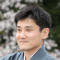Toshiyuki Syo
Principal Application Engineer

## What is e-AI Translator?

A tool that converts learned AI algorithms created using an open-source AI framework into C source code dedicated to inference. The latest version of e-AI Translator, V2.2.0, was released on March 31, 2022. In this blog, we will introduce the new function of V2.2.0 "Function of speeding up by using CMSIS library". This function can be used for the RA family and RX family.

## Concerns About Applying AI to Traditional Programs: Inference Speed

The AI algorithm has polynomial operations with many parameters. Compared to conventional algorithms, the number of parameters and the amount of calculations are large, so the calculation time tends to increase. On the other hand, most of the operation time in this AI algorithm is occupied by the convolution operations. These are calculations that sum the integrated values of a relatively small number of parameters and input data and are easy to speed up.

As an example, let's look at two models used by MLCommons MLPerf™ Tiny Inference benchmark's Keyword Spotting (KWS) and Anomaly Detection (AD), the standard benchmarks for AI for MCUs. Model Source: https://github.com/mlcommons/tiny

Table 1: Neural network functions used in KWS and AD of the MLPerf™ Tiny Inference benchmark standard models and their computation amount

*PW(1x1), DW(3x3): Processing that can be greatly accelerated by using dedicated functions of the CMSIS library.

KWS is a configuration that uses DS-CNN and is mainly composed of Pointwise Convolution and Depthwise Convolution, and AD is AutoEncorder that uses Full Connect. The amount of each calculation is shown in Table 1. In both cases, the functions that make up the majority of operations are covered by the CMSIS library, and it is possible to utilize the DSPs of RX and RA. In particular, KWS uses a dedicated high-speed function, so it can be expected to accelerate the calculation speed.

## Inference Speed Comparison When CMSIS_Int8 Library is Specified

Let's convert the MLPerf™ Tiny Inference benchmark model to C Source by using an e-AI Translator. An Output Format item has been added to the e-AI Translator (Figure 1). Select the library to use in this item.

Figure 1: Library specification (Output Format) when converting the TFLite Int8 model

Let's compare the inference speed and the memory size used when DSP is not used (C_INT8 is specified) and when DSP is used (CMSIS_INT8 is specified). The results are shown in Table 2.

Table 2: Comparison of inference speed and required memory size (GR-KAEDE: RX64M 96MHz, based on inference speed when using C_INT8)

*TFLu+CMSIS: Inference speed when using CMSIS with TensorFlow Lite for Microcomputers (reference value)

In KWS, there are many Convolution layers with high operation costs, and they occupy the majority of inference operations. Therefore, the inference speed is more than 40 times faster due to the effect of speeding up when using the CMSIS library. In the case of AD, the majority of operations are Full Connect layers, which are relatively lightweight. Even if this case, it is more than 10 times faster. On the other hand, due to the temporary buffer array used in the CMSIS function, a maximum RAM size of about twice that of C_INT8 is required.

To use the CMSIS library with RX, please obtain CMSIS for RX. It is a library built to use RX DSP for Arm® CMSIS library. For RA, use FSP3.5 or later, and add CMSIS / DSP and CMSIS / NN in FSP Component. For details on how to use it, refer to the user's manual for e-AI Translator and the application note of CMSIS for RX.